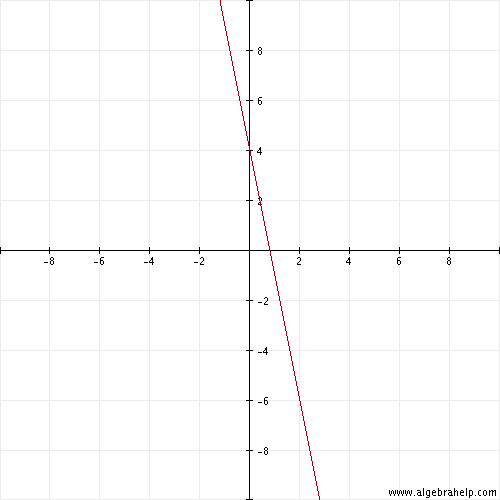f(X)=-5X+4

0  Views: 757 Answers: 2 Posted: 10 years ago
johan vargas

f(X)=-5X+4
chelleanne

Is you graphing? Is you looking for the x and y intercepts?

f (X)= -5X +4

divide both sides of th equation by X

f = -5 + 4

f = -1

f(x) = -5 + 4  is another way in saying..........

y = -5x + 4    >    -5 is the slope and 4 is the y-intercept

GraphBob/PKB

Looks like it passes through at -1. Graphing and intercepts go right over my head
chelleanne

The slope of the line passes through points (0, 4) and (2, -6) I chose those two points because the graph has even numbers. Just give x a value.
-5(2) + 4 = -6 > (2, -6)
-5(0) + 4 = 4 > (0, 4)
Bob/PKB

OK, I see the two places where the line passes. You assigned a value to "X"? Could you have chosen ANY number for "X"?
chelleanne

Yes! This graph have double digits. A single digit graph will have the same outcome. If
x = 1 then y = -1
-5(1) + 4 = -1 > (1, -1)
x = -2 then y = 14
-5(-2) + 4 = 14 > (-2, 14)
x = 3 then y = -11 > (3, -11)
The line will pass through all these points.
Bob/PKB

Thanks for explaining. This is something to refer to again.

### Top contributors in Mathematics category

ROMOS
Karma: 13510

Colleen
Karma: 6890

country bumpkin
Karma: 6240

Bob/PKB
Karma: 5030
> Top contributors chart

Massage Therapy for relief from back pain ?
Answers: 0 Views: 4 Rating: 0
WHAT IS The Chipy?
Answers: 0 Views: 6 Rating: 0
hiii
Answers: 0 Views: 3 Rating: 0
Answers: 0 Views: 6 Rating: 0
why green is green?
Answers: 0 Views: 6 Rating: 0
ไฮโลออนไลน์
Answers: 0 Views: 11 Rating: 0
why red is red?
Answers: 0 Views: 6 Rating: 0
> More questions...

449482
questions
718419